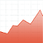V-Lab

## Motivation

Correlations are critical inputs for many financial management tasks. Hedges require esti- mates of the correlation between the returns of assets in the hedge. If the correlations and volatilities are changing, then the hedge ratio should be adjusted to account for the most recent information. Similarly, structured products such as rainbow options that are designed with more than one underlying asset have prices that are sensitive to the correation between the underlying returns. A forecast of future correlations and volatilities is the basis of any pricing formula.

Asset allocation and risk assessment also rely on correlations, however in this case a large number of correlations are often required. Construction of an optimal portfolio with a set of constraints requires a forecast of the covariance matrix of returns. Similarly, the calculation of the standard deviation of today’s portfolio requires a covariance matrix of all the assets in the portfolio. These functions entail estimation and forecasting of large covariance matrices, potentially with thousands of assets.

## Definition

Consider $n$ time series of returns and make the usual assumption that returns are serially uncorrelated. Then, we can define a vector of zero-mean white noises ${\epsilon }_{t}=\mathrm{rt}-\mu$, where $\mathrm{rt}$ is the $n×1$ vector of returns and $\mu$ is the vector of expected returns.

Despite being serially uncorrelated, the returns may present contemporaneous correlation. That is:$∑t=Εt-1[(rt-μ)(rt-μ)′]$may not be a diagonal matrix. Moreover, this contemporaneous variance may be time- varying, depending on past information.

Notice that the elements from the main diagonal of ${\sum }_{t}$ give us conditional variances of the returns, i.e. $\sum _{t}^{\mathrm{i,i}}$ is the conditional variance of the return ${{r}_{t}}^{i}$. Analogously, the elements outside of the main diagonal give us conditional covariances, i.e. $\sum _{t}^{\mathrm{i,j}}$ is the conditional covariance between the returns ${{r}_{t}}^{i}$ and ${{r}_{t}}^{j}$. Hence, we can easily back out the conditional correlations:$Γti,j = ∑ti,j∑ti,i∑tj,j$

## Estimation

The quest for reliable estimates of correlation between return series has motivated much academic and practitioner research. Simple methods such as rolling historical correlations and exponential smoothing are widely used. More complicated models, such as varieties of multivariate GARCH or stochastic volatility, have been extensively investigated in the econometric literature and are used by some sophisticated practitioners. There are many ways to generalize univariate volatility models to the multivariate case, but the curse of dimensionality quickly becomes a major obstacle in applications because there are $k\left(k+1\right)/2$ quantities in ${\sum }_{t}$ for the $k$-dimensional return series. To illustrate, there are 15 conditional variances and covariance in ${\sum }_{t}$ for the five-dimensional return series. In V-Lab, the exponential smoother used by RiskMetrics and several multivariate GARCH models with parsimonious parametrization are considered for correlation analysis.

## References

Engle, R. F. 2009. Anticipating Correlations: A New Paradigm for Risk Management. Princeton University Press.

Tsay, R. S. 2010. Analysis of Financial Time Series. John Wiley & Sons, Inc.